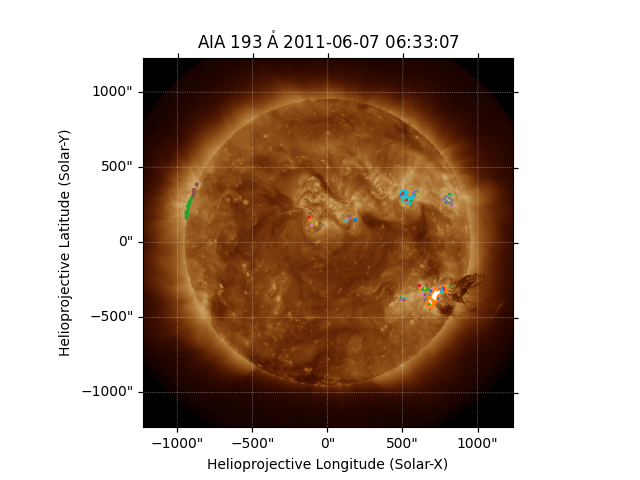# Finding contours of a map¶

This example shows how to find and plot contours on a map.

import matplotlib.pyplot as plt

import astropy.units as u

import sunpy.map
from sunpy.data.sample import AIA_193_IMAGE


aiamap = sunpy.map.Map(AIA_193_IMAGE)


In finding a set of contours, we have to provide the level to contour in the same units as the map data. To find out the units we can inspect sunpy.map.GenericMap.unit.

print(aiamap.unit)

ct


We can see that the units of this map are ct, or counts. We can now chose a contour level, and use the contour() method to extract the contours.

contours = aiamap.contour(50000 * u.ct)


Finally, we can plot the map, and add each of the contours in turn.

fig = plt.figure()